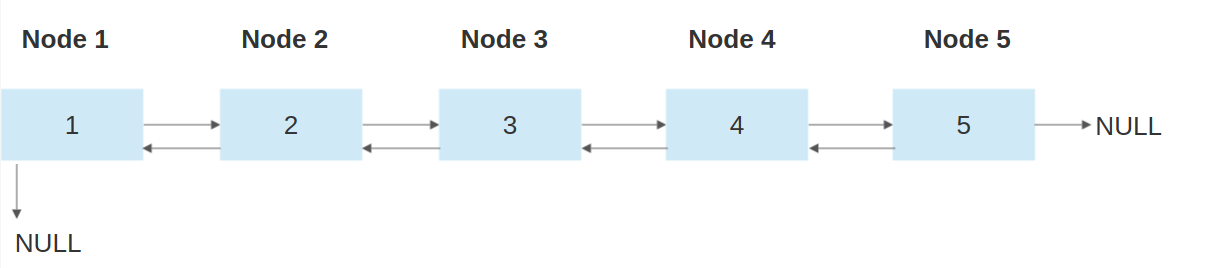×

Search anything:

# Reverse a doubly linked list in C++

#### Algorithms Data Structures linked listGet this book -> Problems on Array: For Interviews and Competitive Programming

In this article, we are going to see how to reverse a doubly linked list by reversing the links of each node (without swapping values of nodes) and have implemented the solution using C++.

## Introduction to Doubly Linked List

A doubly linked list is a linear data structure which is a linked list which contains an extra pointer to store the address of the previous node. It is a variation of singly linked list. In singly linked list, only forward traversal is possible, whereas in doubly linked list, forward and backward traversal is possible.

## Structure of a doubly linked list

A double linked list contains two pointers : a pointer to store the address of the next node (same as in singly linked list) and a pointer to store the address of the previous node. The structure of the doubly linked list also contains a field to store the data of the node.

``````struct node
{
int data;
struct node *nptr; //next pointer
struct node *pptr; //previous pointer
};
``````

## Reversing the doubly linked list

Let us consider a doubly linked list :
Input :Node 1's previous pointer should be NULL and next pointer should point to Node 2

Node 2's previous pointer should point to Node 1 and next pointer should point to Node 3

Node 3's previous pointer should point to Node 2 and next pointer should point to Node 4

Node 4's previous pointer should point to Node 3 and next pointer should point to Node 5

Node 5's previous pointer should point to Node 4 and next pointer should be NULL

Output

We need to reverse both the links of every node of the doubly linked list. That is,
Node 1's previous pointer should point to Node 2 and next pointer be NULL

Node 2's previous pointer points to Node 3 and next pointer points to Node 1

Node 3's previous pointer points to Node 4 and next pointer points to Node 2

Node 4's previous pointer points to Node 5 and next pointer points to Node 3

Node 5's previous pointer points be NULL and next pointer points to Node 4

So, what we would have to do is, first reverse the previous and next pointers of the doubly linked list. We would have to make the previous pointer to point the the next node(instead of the previous node) and the next pointer to point to the previous node(instead of the next node). That's it !
Now let's code it !

## Implementation

Following is the C++ implementation:

``````//Reverse a doubly linked list
#include<iostream>
#include<cstdlib>

using namespace std;

struct node
{
int data;
struct node *nptr; //next pointer
struct node *pptr; //previous pointer
};

void insertNode(int pos, int x)
{
struct node *temp=new node;
if(temp==NULL)
cout<<"Insertion not possible\n";
temp->data=x;
if(pos==1 && hptr==NULL)
{
temp->pptr=NULL;
temp->nptr=NULL;
hptr=temp;
}
else if(pos==1)
{
temp->nptr=hptr;
hptr=temp;
temp->nptr->pptr=temp;
temp->pptr=NULL;
}
else
{
int i=1;
struct node *thptr=hptr;
while(i<pos-1)
{
thptr=thptr->nptr;
i++;
}
temp->nptr=thptr->nptr;
temp->pptr=thptr;
thptr->nptr=temp;
thptr->nptr->pptr=thptr;
}
}

void deleteNode(int pos)
{
if(hptr==NULL)
cout<<"Deletion not possible\n";
else
{

if(pos==1)
{
hptr=hptr->nptr;
}
else
{
int i=1;
struct node *thptr=hptr;
while(pos<i-1)
{
thptr=thptr->nptr;
}
thptr->nptr=thptr->nptr->nptr;
if(thptr->nptr!=NULL)
thptr->nptr->pptr=thptr;
}

}
}

void reverseList()
{
struct node *current=hptr;
struct node *prev=NULL;
while(current!=NULL)
{
current->pptr=current->nptr; //line 1
current->nptr=prev;          //line 2
prev=current;                //line 3
current=current->pptr;       //line 4
}
hptr=prev;
}

void print()
{
struct node *thptr=hptr;
while(thptr!=NULL)
{
cout<<thptr->data<<"\n";
thptr=thptr->nptr;
}
}

int main()
{
insertNode(1,11);
insertNode(2,12);
insertNode(3,13);
insertNode(4,14);
insertNode(5,15);
reverseList();
print();
return 0;
}
``````

OUTPUT :

``````15
14
13
12
11
``````

Let us look at the reverseList() function.
We use 2 pointers, current and prev for the link reversal.
Lines 1,2,3 and 4 are the key in reversing the links of every node.
Line 1 makes the current node's previous pointer point to its next node (link reversal)
Line 2 makes the current node's next pointer point to its previous node(link reversal)
Line 3 updates the prev pointer for link reversal of next node
Line 4 updates the current pointer for link reversal of next node
After every node gets its links reversed, we have one last thing to do :- update the head pointer.
At the end of the while loop, current becomes NULL and prev points to the last node (last node of input list). So in our reversed list, the last node would be the first node, so we update the head pointer as prev
And the doubly linked list is reversed !

## Question 1 :

#### Difference between a doubly linked list and a singly linked list

DLL has an extra pointer
SLL has an extra pointer
Both are the same
None of the options
Doubly Linked lists have an extra pointer to store the address of the previous node. This allows both forward and backward traversal in a doubly linked list.

## Question 2 :

#### How is list reversal different in a singly linked list and a doubly linked list ?

In DLL we have to reverse 2 links for each node
In SLL we did not have to update the head pointer
In SLL we have to reverse 2 links for each node
None of the options
Doubly Linked lists have two links for each node and to reverse the list, we have to reverse both the links#### Harini Jeyaraman

3rd year Computer Science Student at Indian Institute of Information Technology, Design and Manufacturing (IIITDM), Kancheepuram | Intern at OpenGenus

Reverse a doubly linked list in C++